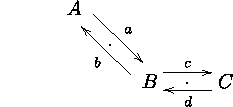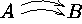## 2.4 Sliding arrows sideways

It is often desirable to have several parallel arrows between two objects. This can be done by sliding either or both arrows sideways a distance given as a TeX dimension enclosed in `@<>`s: it specifies how far "sideways" the arrow should be moved, e.g.,
```\xymatrix{
A \ar@<1ex>[dr]^a_{.} \\
& B \ar@<1ex>[ul]^b \ar@<1ex>[r]^c
& C \ar@<1ex>[l]^d_{.} }```
will typesetA positive distance will slide the arrow in the "`^`-direction," e.g., the two arrows above are slid in the direction of the labels a, b, c, and d, respectively; a negative distance in the "`_`-direction." The distance `<@1ex>` is often appropriate since it corresponds roughly to the height of letters like "x," in the type size being used.

#### Exercise 10.

Typeset.

© Kristoffer H. Rose <krisrose@brics.dk> December 19, 1996 / LaTeX2HTMLv96.1-h